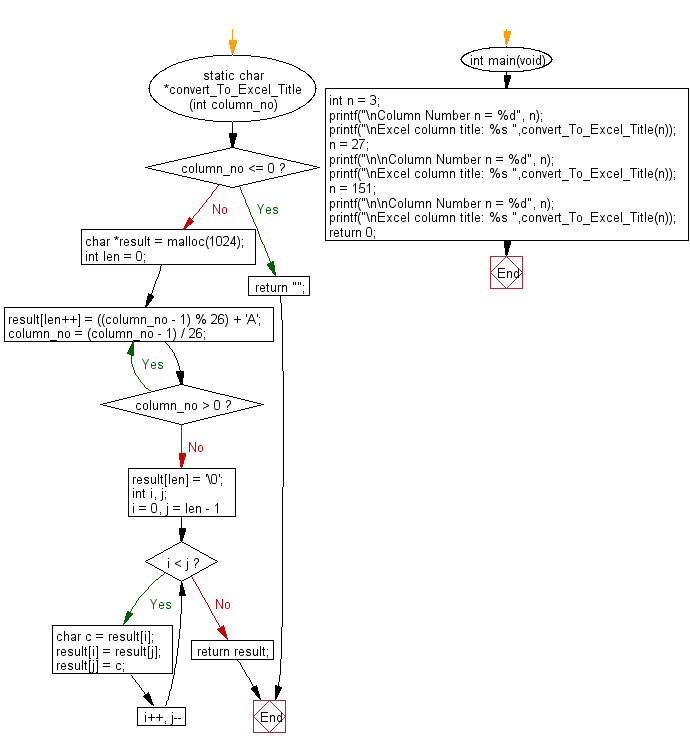﻿ C Program: Excel column title for a given column number

# C Exercises: Get the Excel column title that corresponds to a given column number

## C Programming Mathematics: Exercise-8 with Solution

Write a C program to get the Excel column title that corresponds to a given column number (integer value).

For example:
1 -> A
2 -> B
3 -> C
...
26 -> Z
27 -> AA
28 -> AB
...

Example:
Input:
n = 3
n = 27
n = 151
Output:
Excel column title: C
Excel column title: AA
Excel column title: EU

Pictorial Presentation:Sample Solution:

C Code:

``````#include <stdio.h>
static char *convert_To_Excel_Title(int column_no)
{
if (column_no <= 0) {
return "";
}

char *result = malloc(1024);
int len = 0;
do {
result[len++] = ((column_no - 1) % 26) + 'A';
column_no = (column_no - 1) / 26;
} while (column_no > 0);
result[len] = '\0';

int i, j;
for (i = 0, j = len - 1; i < j; i++, j--) {
char c = result[i];
result[i] = result[j];
result[j] = c;
}
return result;
}

int main(void)
{
int n = 3;
printf("\nColumn Number n = %d", n);
printf("\nExcel column title: %s ",convert_To_Excel_Title(n));
n = 27;
printf("\n\nColumn Number n = %d", n);
printf("\nExcel column title: %s ",convert_To_Excel_Title(n));
n = 151;
printf("\n\nColumn Number n = %d", n);
printf("\nExcel column title: %s ",convert_To_Excel_Title(n));
return 0;
}
```
```

Sample Output:

```Column Number n = 3
Excel column title: C

Column Number n = 27
Excel column title: AA

Column Number n = 151
Excel column title: EU
```

Flowchart:C Programming Code Editor:

Improve this sample solution and post your code through Disqus.

What is the difficulty level of this exercise?

Test your Programming skills with w3resource's quiz.

﻿